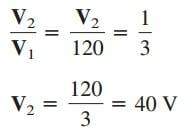# A transformer as an Isolation DeviceHere, we can use transformer as an isolation device. Electrical isolation is said to exist between two devices when there is no physical connection between them.

Contents

In a transformer, energy is transferred by magnetic coupling, without electrical connection between the primary circuit and secondary circuit. We now consider three simple practical examples of how we take advantage of this property.

## Transformer as an Isolation Device

First, consider the circuit in Figure.(1). A rectifier is an electronic circuit that converts an ac supply to a dc supply. A transformer is often used to couple the ac supply to the rectifier. The transformer serves two purposes.

First, it steps up or steps down the voltage.

Second, it provides electrical isolation between the ac power supply and the rectifier, thereby reducing the risk of shock hazard in handling the electronic device.

As a second example, a transformer is often used to couple two stages of an amplifier, to prevent any dc voltage in one stage from affecting the dc bias of the next stage.

Biasing is the application of a dc voltage to a transistor amplifier or any other electronic device in order to produce a desired mode of operation.

Each amplifier stage is biased separately to operate in a particular mode; the desired mode of operation will be compromised without a transformer providing dc isolation.

As shown in Figure.(2), only the ac signal is coupled through the transformer from one stage to the next. We recall that magnetic coupling does not exist with a dc voltage source.

Transformers are used in radio and TV receivers to couple stages of high-frequency amplifiers. When the sole purpose of a transformer is to provide isolation, its turns ratio n is made unity.

Thus, an isolation transformer has n = 1.

As a third example, consider measuring the voltage across 13.2-kV
lines. It is obviously not safe to connect a voltmeter directly to such
high-voltage lines.

A transformer can be used both to electrically isolate the line power from the voltmeter and to step down the voltage to a safe level, as shown in Figure.(3).

Once the voltmeter is used to measure the secondary voltage, the turns ratio is used to determine the line voltage on the primary side.

## Transformer as an Isolation Device Example

Determine the voltage across the load in Figure.(4).Hence, VL2 = 40 V ac or vL2 = 40 cos ωt; that is, only the ac voltage is passed to the load by the transformer. This example shows how the transformer provides dc isolation.